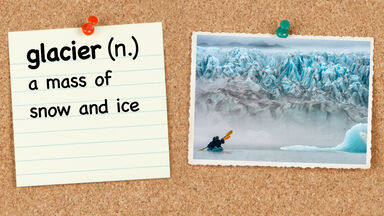# Common 4th Grade Vocabulary Words

Fourth grade is an opportune time to build a student’s vocabulary. But increasing vocabulary doesn’t have to just be a language arts lesson. Keep reading for possible words to add to a fourth grade math, science, social studies, and language arts vocabulary list.4th grade vocabulary word glacier

## Fourth Grade Vocabulary Words With 3-5 Letters

As fourth graders learn more about social studies concepts such as urban and rural areas, they might benefit from these specific vocabulary words. A list of short words also includes math terms such as array, language arts terms such as hero, and science terms such as atom.

• array (n.) - arrangement of numbers that show multiplication problems as repeated addition problems, and division problems as fair shares of a whole
• atom (n.) - the smallest component of an element
• digit (n.) - the expression of numbers 0, 1, 2, 3, 4, 5, 6, 7, 8, or 9
• force (n.) - the agent that causes an item to do work (i.e. move)
• hero (n.) - a person who acts in a courageous or brave way
• mass (n.) - the amount of matter in an object
• ray (n.) - a straight line that extends from a point
• rural (adj.) - relating to living in the country or farmland
• urban (adj.) - relating to living in a large, busy city
• vast (adj.) - immense, stretching
• weary (adj.) - very tired

## Fourth Grade Vocabulary Words With 6-7 Letters

Elementary students are ready to analyze and recognize what they read. But do they know the definitions of these academic terms? Include words with 6-7 letters for both advanced and beginning readers.

• analyze (v.) - to evaluate a situation by splitting it into parts
• ascend (v.) - to go upward
• circuit (n.) - a closed path for electricity to flow
• climate (n.) - weather patterns in a particular area
• decimal (n.) - fraction of a number expressed in base 10
• descend (v.) - to go downward
• divisor (n.) - number that is used to divide another number (dividend)
• eclipse (n.) - an event in which light is blocked by another object
• energy (n.) - the ability to do work
• equator (n.) - the imaginary line between the North and South Poles of the Earth
• erosion (n.) - gradual wearing away of land by water
• errand (n.) - a quick trip to complete a task
• example (n.) - something used as a model for others to follow
• factor (n.) - mathematical expression that is multiplied by another factor
• formula (n.) - standard way to solve a mathematical problem
• fossil (n.) - preserved remains of an ancient or prehistoric organism
• glacier (n.) - a mass of snow and ice
• motion (n.) - movement
• recognize (v.) - to identify someone or something from a previous time
• vertex (n.) - the corner of a shape

## Fourth Grade Vocabulary Words With 8-9 Letters

Math students are busy making sure their dividends and quotients are accurate. Defining these terms, however, may be a trickier task. Try out these interdisciplinary vocabulary words that have 8-9 letters in your next list.

• accurate (adj.) - correct; without errors
• classify (v.) - to sort items into appropriate categories
• coastline (n.) - place where the land meets an ocean boundary
• congruent (adj.) - shapes or figures that have an attribute in common
• diversity (n.) - a population marked by differences
• dividend (n.) - number divided by another number (divisor)
• numerator (n.) - top part of a fraction
• peculiar (adj.) - not like others
• quotient (n.) - the result of dividing one number by another number
• remainder (n.) - number left over in a division problem
• restless (adj.) - constantly moving
• simplify (v.) - to reduce a fraction to its simplest form
• tradition (n.) - a custom passed down from year to year
• variable (n.) - value that could change in a mathematical problem